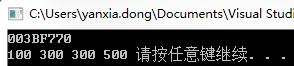# Vector delete pop of element_ back(),erase(),remove()

The member function pop_back() of vector can delete the last element.
The function Erase (), in turn, can remove elements that are indicated by an Iterator, as well as elements of a specified range.
— You can also use the generic algorithm remove() to remove elements in a vector container.
— The difference is: Remove generally does not change the size of the container, whereas member functions such as pop_back() and Erase () do.
1, pop_back ()

`void pop_back();`

Delete last element
Removes the last element in the
vector, effectively reducing the container
size by one.

This destroys the removed element.

``````#include <iostream>
#include <vector>
using namespace std;
int main()
{
vector<int> vec;
int sum(0);
vec.push_back(10);
vec.push_back(20);
vec.push_back(30);
while(!vec.empty())
{
sum += vec.back();
vec.pop_back();
}
cout<<"vec.size()="<<vec.size()<<endl;
cout<<"sum = "<<sum<<endl;
system("pause");
return 0;
}``````

0

60
2、erase()
C++98

```iterator erase (iterator position);
iterator erase (iterator first, iterator last);
```

C++11

```iterator erase (const_iterator position);
iterator erase (const_iterator first, const_iterator last);```

Deletes an element in the specified location or deletes an element in the specified range
Removes from the vector of either a single element (position) or a range of elements ( [first, last) .) including the first, not including the last.

This effectively reduces the container size by the number of elements removed, which are destroyed.
It reduces the size of the container. After the iterator is used on the erase element, it subsequently fails, i.e., the iterator can no longer operate on the vector.

``````#include <iostream>
#include <vector>
using namespace std;
int main()
{
vector<int> vec;
for(int i=0;i<10;i++)
{
vec.push_back(i);
}
vec.erase(vec.begin()+5);//erase the 6th element
vec.erase(vec.begin(),vec.begin()+3);
for(int i=0;i<vec.size();i++)
{
cout<<vec[i]<<' ';
}
cout<<endl;
system("pause");
return 0;
}``````

// Output 3, 4, 6, 7, 8, 9
3. Remove () Not recommended

``````#include <iostream>
#include <vector>
using namespace std;
int main()
{
vector<int> vec;
vec.push_back(100);
vec.push_back(300);
vec.push_back(300);
vec.push_back(300);
vec.push_back(300);
vec.push_back(500);
cout<<&vec<<endl;
vector<int>::iterator itor;
for(itor=vec.begin();itor!=vec.end();itor++)
{
if(*itor==300)
{
itor=vec.erase(itor);
}
}
for(itor=vec.begin();itor!=vec.end();itor++)
{
cout<<*itor<<" ";
}
system("pause");
return 0;
}``````